# 解 SSA 三角形

"SSA" 的意思是 "(S)ide, (S)ide, (A)ngle"（英语 "Angle" 的意思是 角，"Side" 的意思是 边）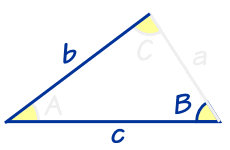"SSA" 的意思是我们知道两个边长和一个角度，（而这个角不是 在已知的两条边之间）。

 解 SSA 三角形 先用 正弦定理 来求未知的两个角； 再用 三个角的和是 180° 来求剩下的角； 最后用正弦定理来求未知边长。

### 例 1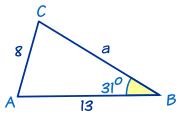• 边 b = 8
• 边 c = 13

sin(C)/c = sin(B)/b
sin(C)/13 = sin(31°)/8
sin(C) = (13×sin(31°))/8
sin(C) = 0.8369……
C = sin−1(0.8369……)
C = 56.818……°
C = 56.8° 准确到1个小数位（*看下面）

A = 180° − 31° − 56.818……°
A = 92.181……° = 92.2° 准确到1个小数位

a/sin(A) = b/sin(B)
a/sin(92.181……°) = 8/sin(31°)

a = (sin(92.181……°) × 8)/sin(31°)
a = 15.52 准确到2个小数位

…… 真的解了？

* 在上面计算：

C = sin−1(0.8369……)
C = 56.818……°

C 的另外一个答案是 180° − 56.818……°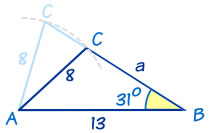C = 180° − 56.818……°
C = 123.2° 准确到1个小数位

C 的值不同，角 A 和 边 a的值也不同

A = 180° − 31° − 123.181……°
A = 25.818……°
A = 25.8° 准确到1个小数位

a/sin(A) = b/sin(B)
a/sin(25.818……°) = 8/sin(31°)
a = (sin(25.818……°)×8)/sin(31°)
a = 6.76 准确到2个小数位

C =  56.8°、A = 92.2°、a = 15.52

C = 123.2°、A = 25.8°、a = 6.76

### 例 2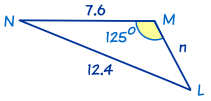sin(L)/l = sin(M)/m
sin(L)/7.6 = sin(125°)/12.4
sin(L) = (7.6×sin(125°))/12.4
sin(L) = 0.5020……
L = 30.136……°
L = 30.1° 准确到1个小数位

N = 180° − 125° − 30.136……°
N = 24.863……°
N = 24.9° 准确到1个小数位

n/sin(N) = m/sin(M)
n/sin(24.863……°) = 12.4/sin(125°)
n = (sin(24.863……°)×12.4)/sin(125°)
n = 6.36 准确到2个小数位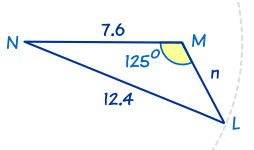L 的另外一个可能答案是 149.9°。但在这例子这是不可能的，因为已经有 M = 125°，而一个三角形不可以有两个角大于 90°。

## 结论：

"Side, Side, Angle"（SSA）三角形时，我们需要